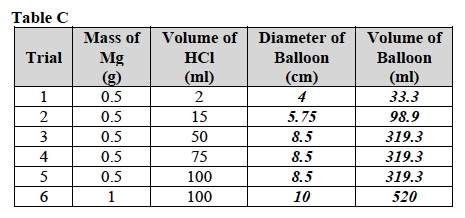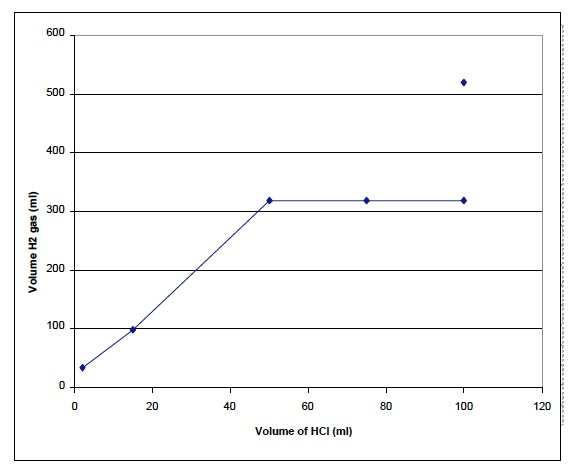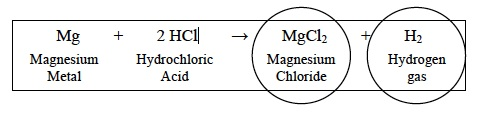# Chemical Reactions: Investigation 2 PostLabZERO-IN

Italicized font represents information to be shared orally or physically completed with the students at this time.

Non-italicized font represents additional information included to support the teacher’s understanding of the content being introduced within the CELL.

ANALYZE IT

When students have finished their Investigation, discuss the data in Table C.

Note: For each Trial, students should use the measured diameter of the inflated balloon to calculate the volume of hydrogen gas produced.

Direct students to the Analysis section in their Student Data Record for instructions on using the formula for the volume of a sphere.

Note: Students should calculate the volume of the inflated balloon for every Trial and enter the volumes in Table C.Ask students to complete the analysis of their data by graphing their data from Table C in their Student Data Record using the procedure for Graphing of Independent and Dependent Variables.

Note: Students should connect the data points for Trials 1 through 5 with straight lines and not draw a best fit line.

Note: Students should plot the data point from Trial 6 and not draw a line through it.Ask students to analyze the graph in the range of hydrochloric acid volumes between 2 and 50 ml then answer the questions in problems 3 and 4 of the Analysis section in their Student Data Record.

• What is the relationship between the volume of hydrochloric acid added as a reactant and the volume of hydrogen gas (H2) that was produced as a product? Student answers will vary. For every increase in the volume of hydrochloric acid, the volume of hydrogen gas increases.
• In this range of hydrochloric acid volume, do you think that the hydrochloric acid or the magnesium is greater in amount? Why? (Hint: Remember that you looked into the flask after Trials 1-3 were complete to observe if any magnesium remained.) Student answers will vary. In this range of hydrochloric acid (HCl) volume, the magnesium metal (Mg) is in greater amount because magnesium metal (Mg) was observed to remain after the reactions were complete.

Ask students to analyze the graph in the range of hydrochloric acid (HCl) volumes between 50 and 100 ml then answer the questions in their Student Data Record.

• What is the relationship between the volume of hydrochloric acid added as a reactant and the volume of hydrogen gas that was produced as a product? Student answers will vary. For every increase in the volume of hydrochloric acid used as a reactant, the volume of hydrogen gas remained the same.
• In this range of volumes of hydrochloric acid, do you think that the hydrochloric acid or the magnesium is greater in amount? Why? (Hint: Remember that you looked into the flask after Trials 3-5 were complete to observe if any magnesium remained.) Student answers will vary. In this range of hydrochloric acid volume, the hydrochloric acid is in greater amount because no magnesium was observed to remain after the reactions were complete.

Ask students to read the Background in their Student Data Record and discuss the case where one reactant is present in a greater amount than the other.

Ask the students about a chemical reaction in which one reactant remains unreacted. Ask students to answer the questions in their Student Data Record.

• If one of the reactants is completely consumed but some of the second reactant remains unreacted, which reactant limits the amount of products that can be produced? Why? Student answers will vary. The reactant that is completely consumed limits the amount of the products that can be produced because there is no more of that reactant left.
• Analyze the graph in the range of hydrochloric acid volumes between 50 and 100 ml. Why did the diameter of the balloon and the volume of hydrogen gas remain unchanged in this range of hydrochloric acid volumes? Student answers will vary. The magnesium was completely consumed in this range of hydrochloric acid volumes because the hydrochloric acid was present in a greater amount.

Ask students to analyze the graph for the two data points for the reactions of 0.5 g of magnesium and 1 g of magnesium metal with 100 ml of hydrochloric acid. Ask students to answer the questions in their Student Data Record.

• Compare the volume of hydrogen produced by the reactions of 0.5 g and 1 g of magnesium with 100 ml of hydrochloric acid. Which reaction produced a greater volume of hydrogen H2? Student answers will vary. The reaction using 1 g of magnesium produced the greater volume of hydrogen gas.
• Why did one reaction produce more hydrogen H2 gas than the other? Student answers will vary. More hydrogen was produced in the reaction using 1 g of magnesium because unreacted hydrochloric acid remained.
• In the reaction of 100 ml of hydrochloric acid and 0.5g of magnesium, which reactant was present in the lesser amount? Student answers will vary. The magnesium was present in the lesser amount because the 0.5 g of magnesium was used up. When a greater amount of magnesium, 1 g, was added to 100 ml of hydrochloric acid, more hydrogen gas was produced suggesting that an excess of hydrochloric acid remained after the 0.5 g of magnesium was used up.

Write the chemical equation on the board and ask students to complete number 12 in their Student Data Record.

• What are the two products of the chemical reaction that you have observed? Circle the products Magnesium chloride (MgCl2) and hydrogen gas (H2).Based on the data collected in the Investigation, ask students to answer the following questions and compare their conclusions with their predicted answers.

• Are the reactants always completely used up in a chemical reaction? If the reactants are present in equal amounts, then each reactant will be completely used up.
• Does the matter making up the reactants disappear? The matter making up the reactants does not disappear, it is found in the products of the reaction.
• What happens if there is more of one reactant than the other reactant? If there is more of one reactant than the other reactant, then it will not be completely used up in the reaction.
• How do you know when a chemical reaction has stopped? No more products are produced when a chemical reaction has stopped.

GET FOCUSED

Instruct students to complete the Focus Questions in their SDRs then discuss them as a class. Use the suggested responses below to guide students’ answers.

• In a chemical reaction, do the amounts of the reactants affect the amounts of products produced? Student answers will vary. Reactants must be present in the reaction in equivalent amounts to produce the maximum amount of all products.
• How does the Law of Conservation of Matter relate to chemical reactions? Student answers will vary. No matter is lost from a chemical reaction even if the reactants are present in amounts that are not equivalent. Every atom of every reactant can be found in either the products or in reactants that are not consumed.